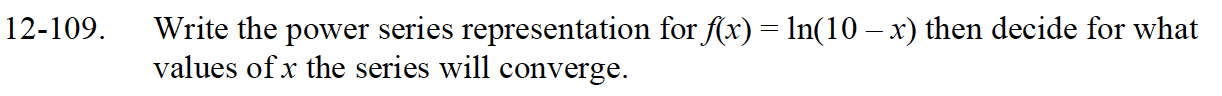### Home > CALC > Chapter 12 > Lesson 12.3.1 > Problem12-109

12-109.

Write the power series representation for f(x) = ln(10 – x) then decide for what values of x the series will converge. Homework Help ✎$\text{The power series for }f(x)\text{ is }\int-\frac{1}{10}\Big(1+\frac{x}{10}+\big(\frac{x}{10}\big)^2+...\Big)dx.$

$\text{Ratio Test: } \lim_{n\to\infty}\Bigg|\frac{\Big(\frac{x}{10}\Big)^{n+2}\Big(\frac{1}{n+1}\Big)}{\Big(\frac{x}{10}\Big)^{n+1}\Big(\frac{1}{n}\Big)}\Bigg|$